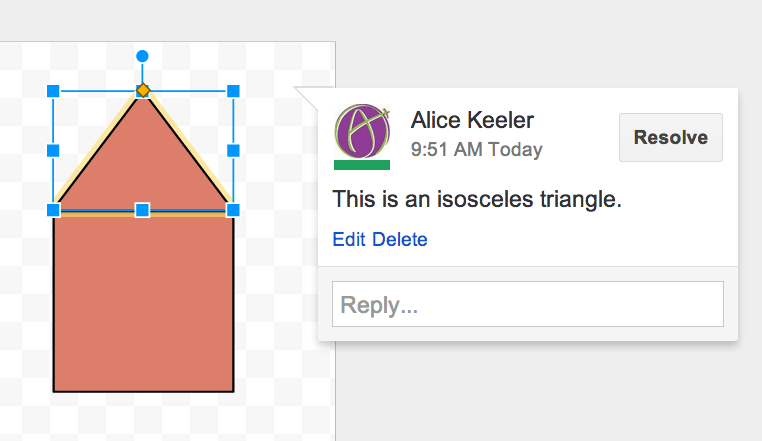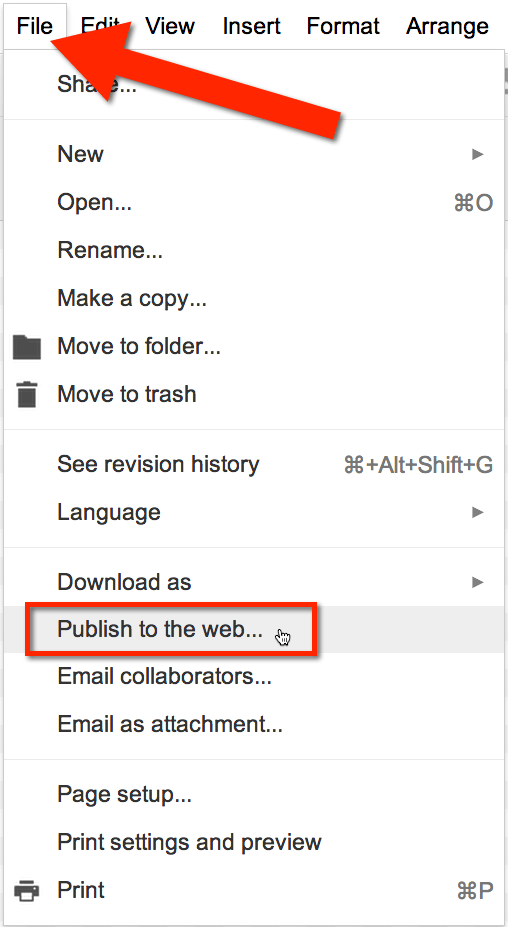CCSS.MATH.PRACTICE.MP4 Model with mathematics.
Mathematically proficient students can apply the mathematics they know to solve problems arising in everyday life, society, and the workplace. In early grades, this might be as simple as writing an addition equation to describe a situation. In middle grades, a student might apply proportional reasoning to plan a school event or analyze a problem in the community. By high school, a student might use geometry to solve a design problem or use a function to describe how one quantity of interest depends on another. Mathematically proficient students who can apply what they know are comfortable making assumptions and approximations to simplify a complicated situation, realizing that these may need revision later. They are able to identify important quantities in a practical situation and map their relationships using such tools as diagrams, two-way tables, graphs, flowcharts and formulas. They can analyze those relationships mathematically to draw conclusions. They routinely interpret their mathematical results in the context of the situation and reflect on whether the results make sense, possibly improving the model if it has not served its purpose.

One of the mathematical practices for the Common Core is to “Model with mathematics.” Google Drive has a tool to allow students to visually model their mathematics: Google Draw.

Earlier I had blogged about using Google Draw for virtual manipulatives. This can help students to model their mathematics with pre-designed manipulatives. However, if we want students to think critically they need to be able to choose the appropriate way of modeling their understanding, which means we do not give them a template.

CCSS.MATH.PRACTICE.MP5 Use appropriate tools strategically.
Mathematically proficient students consider the available tools when solving a mathematical problem. These tools might include pencil and paper, concrete models, a ruler, a protractor, a calculator, a spreadsheet, a computer algebra system, a statistical package, or dynamic geometry software. Proficient students are sufficiently familiar with tools appropriate for their grade or course to make sound decisions about when each of these tools might be helpful, recognizing both the insight to be gained and their limitations. For example, mathematically proficient high school students analyze graphs of functions and solutions generated using a graphing calculator. They detect possible errors by strategically using estimation and other mathematical knowledge. When making mathematical models, they know that technology can enable them to visualize the results of varying assumptions, explore consequences, and compare predictions with data. Mathematically proficient students at various grade levels are able to identify relevant external mathematical resources, such as digital content located on a website, and use them to pose or solve problems. They are able to use technological tools to explore and deepen their understanding of concepts.

In the new Google Drive the Google Draw can be found by going to “New” and choosing “More” down at the bottom.

## Shift D

Teach students that once they are in Google Drive to hold down the shift key and strike the D key. This will create a Google Drawing without having to use the “New” button.

# Collaborative

Like all Google documents, Google Drawings are collaborative. The blue share button in the upper right hand corner allows the student to share the Drawing with the teacher, or with other students. One of the 4 C’s of the Common Core is collaboration. Try having 2 students collaborate on a single Google Drawing to model their mathematics.Like other Google Documents, you can insert comments. Click on an element (or select a group of elements by holding down the shift key as you select) and right click to insert a comment. The keyboard shortcut of Control Alt M also works to insert a comment. This allows students to not only model their mathematics but to explain and label the elements of their model. As the teacher you are able to reply to the comments to help give students feedback and ask the students questions to deepen their understanding of the concepts.# Embed

If you are having students maintain a digital portfolio of their work, the Google Drawings can be embedded into the student websites. Students can go to File->Publish to the web… to obtain the embed code of their drawing. The best part is the embedded drawing is always updated. As the student responds to feedback and makes improvements to their model, their digital portfolio will automatically reflect the changes. Note that when embedding a Google Doc the comments do not publish. This allows the students conversations with the instructor to remain private, but the drawing to be be published.## Subscribe to the Alice Keeler Newsletter